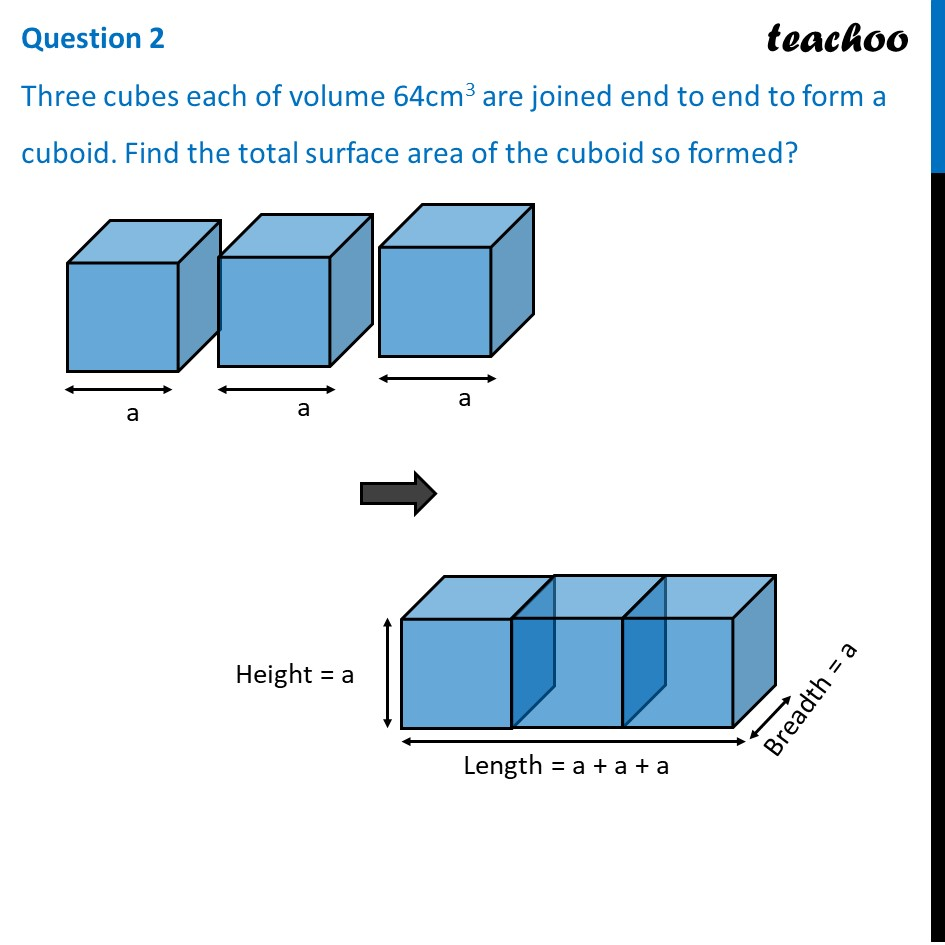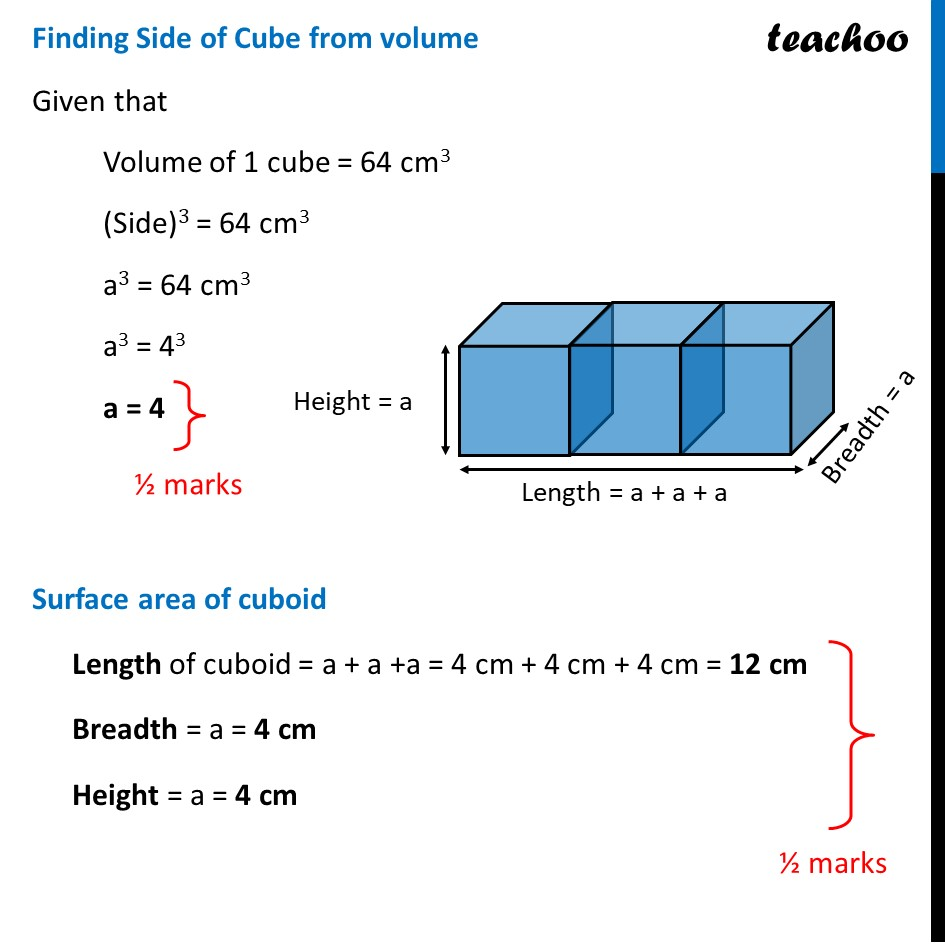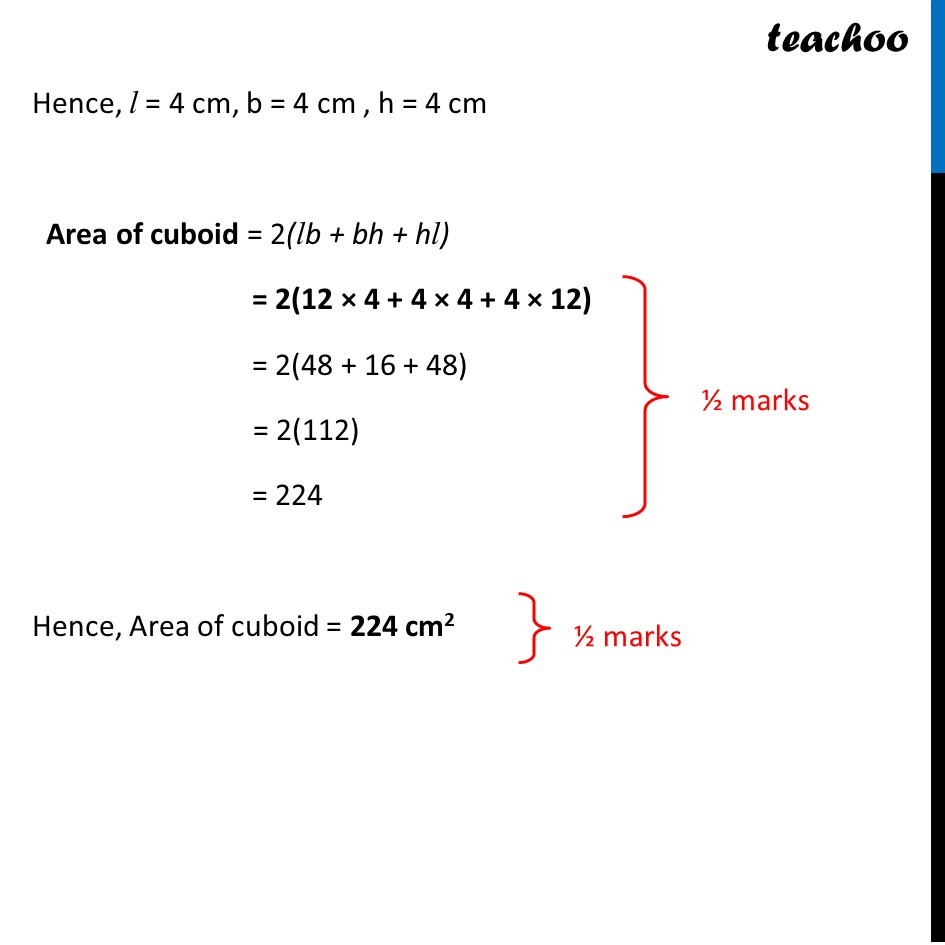CBSE Class 10 Sample Paper for 2022 Boards - Maths Basic [Term 2]

Class 10
Solutions of Sample Papers for Class 10 Boards

## Three cubes each of volume 64 cm 3 are joined end to end to form a cuboid. Find the total surface area of the cuboid so formed?

This question is similar to Ex 13.1, 1 Chapter 13 Class 10 - Quadratic EquationsLearn in your speed, with individual attention - Teachoo Maths 1-on-1 Class

### Transcript

Question 2 Three cubes each of volume 64cm3 are joined end to end to form a cuboid. Find the total surface area of the cuboid so formed? Finding Side of Cube from volume Given that Volume of 1 cube = 64 cm3 (Side)3 = 64 cm3 a3 = 64 cm3 a3 = 43 a = 4 Surface area of cuboid Length of cuboid = a + a +a = 4 cm + 4 cm + 4 cm = 12 cm Breadth = a = 4 cm Height = a = 4 cm Hence, l = 4 cm, b = 4 cm , h = 4 cm Area of cuboid = 2(lb + bh + hl) = 2(12 × 4 + 4 × 4 + 4 × 12) = 2(48 + 16 + 48) = 2(112) = 224 Hence, Area of cuboid = 224 cm2